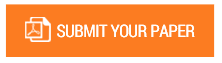Study of Water Discharge Statistic and Arithmetic Flows using Awlr in Jeneberang River in Gowa

Authors : Ratna Musa; Syarifa Tenriola; Melinda Mispa; Trifandy M.W

Volume/Issue : Volume 6 - 2021, Issue 12 - December

Scribd : https://bit.ly/3sTq0lM

Bili-bili dam is located in the upper part of the Jeneberang river where in this area there is an automatic water level measuring device or AWRL (Automatic Water Level Recorder). Continuous river discharge monitoring is very necessary to evaluate the watershed in the long term. The method used in monitoring the discharge is the discharge curve method or rating curve. The use of flow rate curve data that is not up to date can cause an error in the estimation of flood discharge. The purpose of this study is to find out how much is the debit and the percentage difference from the Rating Curve equation generated through the statistical and arithmetic methods. The method used in determining the price of H0 is Arithmetic. The results of this study conclude that the rating curve equation in 2009-2015 statistic method is Q = 3,335 X (H-83,67) 0,98 in 2016-2019 is Q = 5,898 X (H-88,67) 0,86 while arithmetic method in 2009-2015 is Q = 5,419 X (H-83,67) 1 in 2016-2019 is Q = 8,033 X (H-88,67) 1 and the difference in the value of river discharge and calculated discharge with statistic method in 2009-2015 an average of -3,036% in 2016-2019 an average of -1,68% while in arithmetic method in 2009-2015 an average of 72,13% in 2016-2019 an average of -71,65%. The result of this study are expected to be a reference method that can be used to calculated river discharge in other areas.

Keywords : Automatic Water Level, Rating Curve, River.

#### CALL FOR PAPERS

Paper Submission Last Date
30 - June - 2022